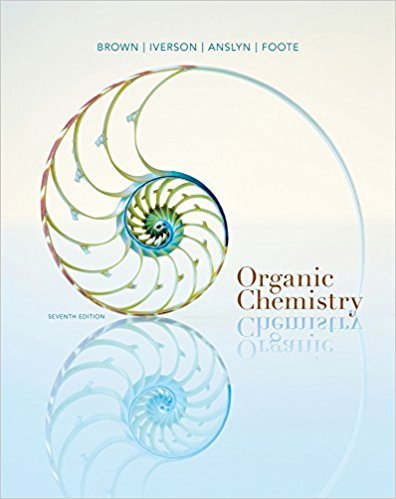×
Get Full Access to Organic Chemistry - 7 Edition - Chapter 11 - Problem 11.34
Get Full Access to Organic Chemistry - 7 Edition - Chapter 11 - Problem 11.34

×

# Show reagents to convert bromocyclopentane to each of the following compoundsISBN: 9781133952848 483

## Solution for problem 11.34 Chapter 11

Organic Chemistry | 7th Edition

• Textbook Solutions
• 2901 Step-by-step solutions solved by professors and subject experts
• Get 24/7 help from StudySoup virtual teaching assistantsOrganic Chemistry | 7th Edition

4 5 1 309 Reviews
12
3
Problem 11.34

Show reagents to convert bromocyclopentane to each of the following compounds.

Step-by-Step Solution:
Step 1 of 3

Lecture Notes week 4: 9/26­9/30  Can use mass/mole conversions to predict the amount of products in a reaction from a given amount of reactant  Procedure for Stoichiometric calculations 1. Write a balanced equation. You get the stoichiometric coefficients this way. 2. Draw a map of starting a finishing points 1 Write out calculations with units. 2 Calculate  Limiting Reagents: amount of one of the reagents (reactants) limits how much product can be formed. o Calculate the amount of product possible from each of the given amounts of reactants, the smallest amount is from the limiting reagent  Percent Yield = (actual amount produced/theoretical amount calculated)*100%

Step 2 of 3

Step 3 of 3

## Discover and learn what students are asking

Calculus: Early Transcendental Functions : Differentiation and Integration of Vector-Valued Functions
?Differentiation of Vector-Valued Functions In Exercises 1-6, find r’(t), $$\mathrm{r}\left(t_{0}\right)$$, and $$\mathrm{r’}\left(t_{0}\right)$$ for t

Statistics: Informed Decisions Using Data : Inference about the Difference between Two Medians: Dependent Samples
?Car Rentals The following data represent the weekday rental rate for a compact car charged by two car-rental companies, Avis and Hertz, in 11 location

Unlock Textbook Solution